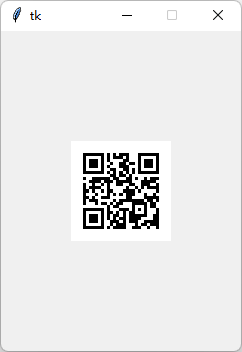# 5 - 基础控件

## |- 5.1-文字 draw_text

• 返回值： 文字对象

• 输入参数：

• x : 横坐标
• y : 纵坐标
• w : 长度，如果超出长度则自动换行，此参数可省略。
• text ： 要显示的文字
• color : 文字颜色
• origin : 对齐位置，默认左上角
• onclick : 当被点击时触发的回调函数
• font_family : 指定字体
• font_size : 文字大小
• angle :文字旋转角度
• 用法举例（代码）：

``````import time
from unihiker import GUI   #导入包
gui=GUI()  #实例化GUI类
def info_text_on_click():
print("文字被点击")

info_text = gui.draw_text(x=120, y=320, text='你好',origin='bottom' ,onclick=info_text_on_click)

info_text2 = gui.draw_text(x=0, y=100, text='1234567890')
info_text3 = gui.draw_text(x=0, y=150, w=50, text='1234567890')#换行演示

# 加载自己下载的字库文件,输入参数为本地字库文件所在路径
# 字库下载参考网站：https://www.fontspace.com/category/led
#gui.draw_text(x=20, y=80, text='13:11', font_size=40, font_family=font_family) #使用字库显示

while True:
#增加等待，防止程序退出和卡住
time.sleep(1)
``````
• 用法举例（图形化）：

unihiker库的画图控件均使用对象方式更新，因此需要给每个控件定一个对象名字，后续使用对象名调整功能。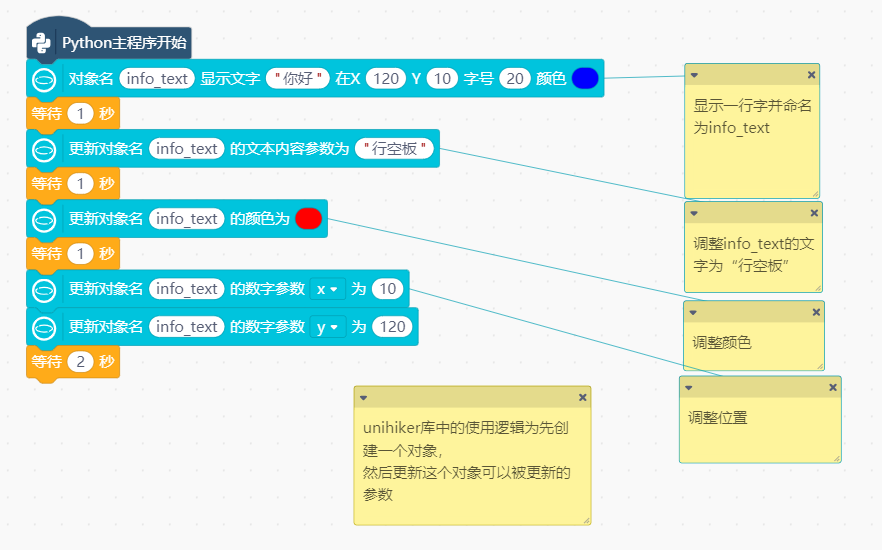• 效果截图：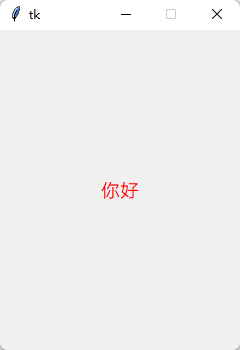## |- 5.2-数码管字体 draw_digit

• **返回值：**数码管对象

• 输入参数：

• x : 横坐标
• y : 纵坐标
• text ： 要显示的文字
• color : 文字颜色
• origin : 对齐位置，默认左上角
• onclick : 当被点击时触发的回调函数
• font_size : 文字大小
• 用法举例：

``````from unihiker import GUI   #导入包
gui=GUI()  #实例化GUI类

gui.draw_digit(x=120, y=160, text='2022-02-01', origin = "center",color="red",font_size=25)#数码管字体显示

import time
while True:
#增加等待，防止程序退出和卡住
time.sleep(1)

``````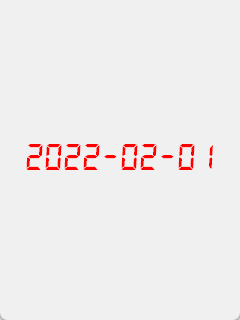## |- 5.3-图片 draw_image

• **返回值：**图片对象
• 输入参数：
• x : 横坐标
• y : 纵坐标
• w : 图片的宽度。按照长宽最小边等比例缩放，可省略，宽高参数都省略则保持原图分辨率。
• h : 图片的高度。按照长宽最小边等比例缩放，可省略，宽高参数都省略则保持原图分辨率。
• image : 图片源，可以传入路径或image对象
• origin : 对齐位置，默认左上角
• onclick : 当被点击时触发的回调函数
• 用法举例：
``````#  -*- coding: UTF-8 -*-
from unihiker import GUI   #导入包
gui=GUI()  #实例化GUI类

# 传入图片文件路径的方式
## 直接传入图片，原始尺寸
img_image2 = gui.draw_image(x=0, y=0, image='logo.png')
## 修改尺寸
img_image = gui.draw_image(x=120, y=100, w=80, h=50,  image='logo.png', origin='center', onclick=lambda: print("image clicked"))

# 使用PIL库传入图片对象的方式
from PIL import Image
img_image3 = gui.draw_image(x=10, y=180, image= Image.open('logo.png'))

import time
while True:
#增加等待，防止程序退出和卡住
time.sleep(1)
``````
• 用法举例（图形化）：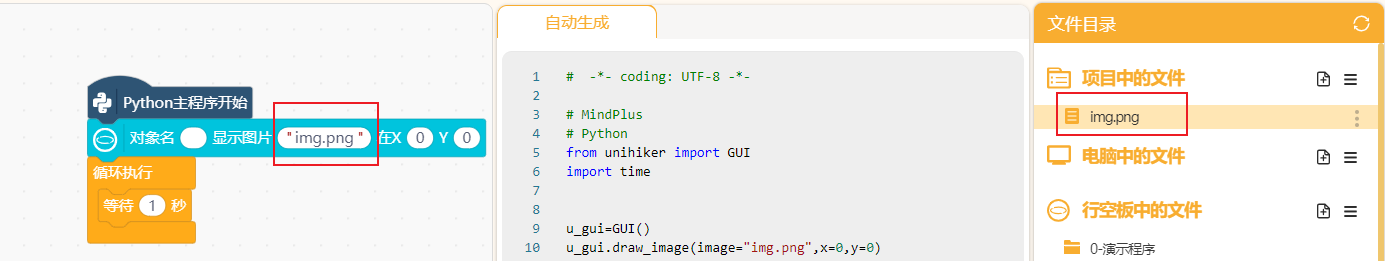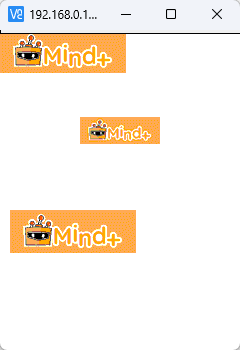## |- 5.3-表情 draw_emoji

• **返回值：**表情对象
• 输入参数：
• x : 横坐标
• y : 纵坐标
• w : 表情的宽度。按照长宽最小边等比例缩放，可省略，宽高参数都省略则保持原图分辨率。
• h : 表情的高度。按照长宽最小边等比例缩放，可省略，宽高参数都省略则保持原图分辨率。
• emoji : 表情名，可以使用内置表情（Angry,Nerve,Peace,Shock,Sleep,Smile,Sweat,Think,Wink）也可以传入路径表情图片（按照`名字-序号.png`格式）
• duration : 图片切换间隔时间
• origin : 对齐位置，默认左上角
• onclick : 当被点击时触发的回调函数
• 用法举例：
``````from unihiker import GUI   #导入包
import time
gui=GUI()  #实例化GUI类

emj1 = gui.draw_emoji(x=0, y=0, w=100, h=100, emoji="Wink", duration=0.1, onclick=lambda:cb("emojis clicked"))
emj2 = gui.draw_emoji(x=120, y=200, w=100, h=100, emoji="Smile", duration=1,origin="center" ,
onclick=lambda:cb("emojis clicked"))
# 传入图片文件路径的方式，同级目录下放多帧图片文件，custom-1.png、custom-2.png、custom-3.png...
# gui.draw_emoji(x=0, y=0, w=100,  emoji="custom", duration=0.2)

while True:
time.sleep(1) #等待防止程序退出看不到效果
``````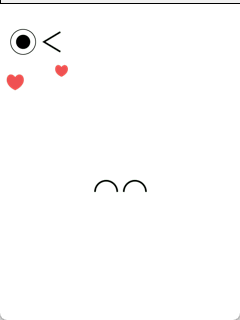• **返回值：**按钮对象
• 输入参数：
• x : 横坐标
• y : 纵坐标
• w : 图片的宽度
• h : 图片的高度
• text : 按钮上显示的文字
• origin : 对齐位置，默认左上角
• state : 按钮使能，设置为 `disabled` 时按钮无法点击，设置为 `normal` 则回复到正常可点击状态
• onclick : 当被点击时触发的回调函数。注意函数中勿执行需要长时间运行的程序，否则会卡住按钮，可以启动一个新的线程来执行需要耗费较长时间的程序。
• 用法举例：
``````from unihiker import GUI   #导入包
gui=GUI()  #实例化GUI类
gui.add_button(x=120, y=110, w=100, h=30, text="按钮", origin='center', onclick=lambda: print("button clicked"))
gui.add_button(x=120, y=210, w=100, h=30, text="按钮", origin='center', onclick=lambda: print("button clicked"),state="disabled")

import time
while True:
#增加等待，防止程序退出和卡住
time.sleep(1)
``````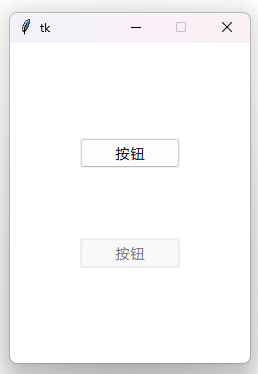``````#多个按钮调用同一个函数并传参示例
from unihiker import GUI   #导入包
gui=GUI()  #实例化GUI类

def btclick(data):
print(data)
txt.config(text=str(data))

txt=gui.draw_text(text="0",x=120,y=10,font_size=20,origin="center",color="#0000FF")

gui.add_button(x=120, y=100, w=100, h=30, text="按钮1", origin='center', onclick=lambda: btclick(1))
gui.add_button(x=120, y=150, w=100, h=30, text="按钮2", origin='center', onclick=lambda: btclick(2))
gui.add_button(x=120, y=200, w=100, h=30, text="按钮3", origin='center', onclick=lambda: btclick(3))

import time
while True:
#增加等待，防止程序退出和卡住
time.sleep(1)
``````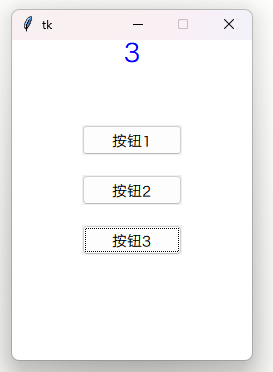• 用法举例（图形化）：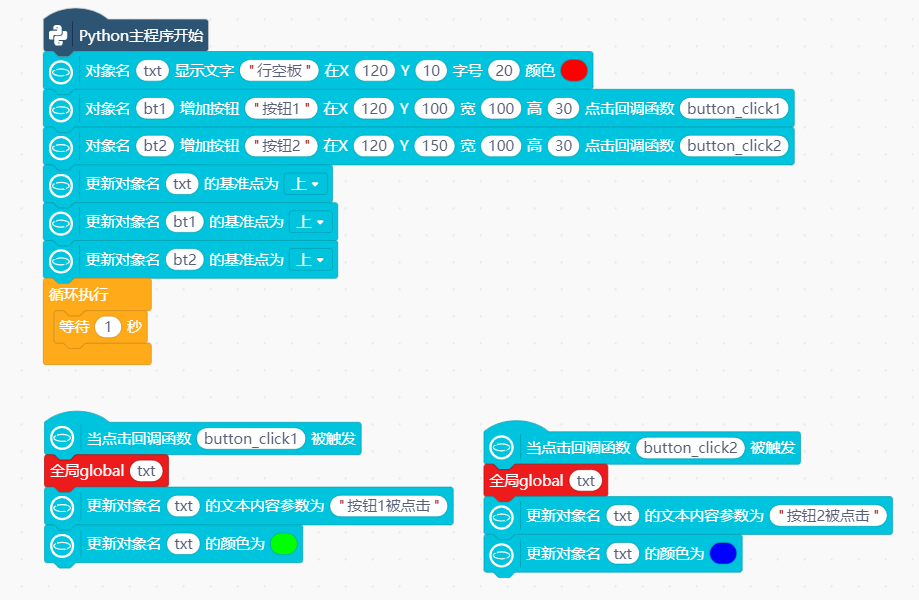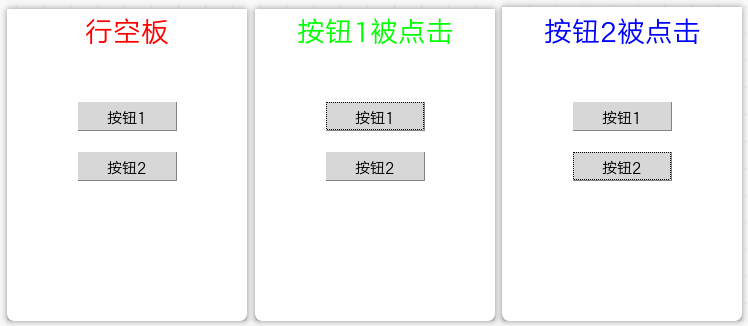## |- 5.5-时钟 draw_clock

• **返回值：**时钟对象
• 输入参数：
• x : 横坐标
• y : 纵坐标
• r : 半径
• h : 时
• m : 分
• s : 秒
• color : 边框颜色
• origin : 对齐位置，默认左上角
• onclick : 当被点击时触发的回调函数
• 用法举例：
``````from unihiker import GUI   #导入包
gui=GUI()  #实例化GUI类
#创建时钟对象
clk = gui.draw_clock(x=120, y=160, r=80, h=10, m=8, s=0, color="#0000FF", onclick=lambda: print("clock clicked"))

import time
import datetime
while True:
#从datetime库中获取系统时分秒，更新到时钟对象中实现走时
#行空板联网后系统时间自动校准
clk.config(h=datetime.datetime.now().hour,m=datetime.datetime.now().minute,s=datetime.datetime.now().second)

#增加等待，防止程序退出和卡住
time.sleep(1)
``````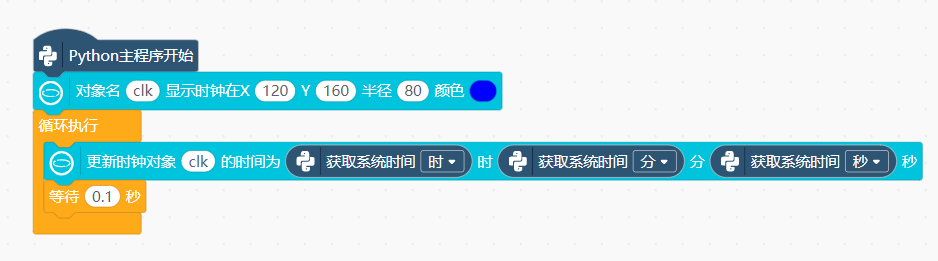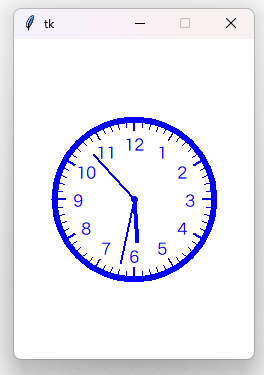## |- 5.6-填充时钟 fill_clock

• **返回值：**填充时钟对象
• 输入参数：
• x : 横坐标，默认中心对齐
• y : 纵坐标
• r : 半径
• h : 时
• m : 分
• s : 秒
• color : 边框颜色
• fill : 填充颜色
• style : 预设模式，当前仅有两种，dark和light
• onclick : 当被点击时触发的回调函数
• 用法举例：
``````from unihiker import GUI   #导入包
gui=GUI()  #实例化GUI类
gui.fill_clock(x=120, y=100, r=60, h=3, m=4, s=5, color=(255, 255, 255), fill="#57b5ff", onclick=lambda: print("clock1 clicked"))
gui.fill_clock(x=120, y=230, r=60, h=6, m=7, s=8, style='dark', onclick=lambda: print("clock2 clicked"))

import time
while True:
#增加等待，防止程序退出和卡住
time.sleep(1)
``````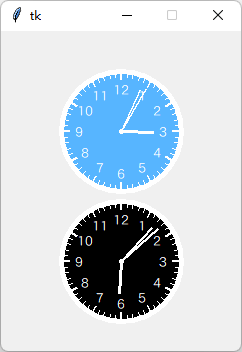## |- 5.7-二维码 draw_qr_code

• **返回值：**二维码对象
• 输入参数：
• x : 横坐标
• y : 纵坐标
• w : 二维码的边长
• text : 二维码扫描后得到的内容
• origin : 对齐位置，默认左上角
• onclick : 当被点击时触发的回调函数
• 用法举例：
``````from unihiker import GUI   #导入包
gui=GUI()  #实例化GUI类
gui.draw_qr_code(x=100, y=200, w=100, text="https://unihiker.com", origin ="center",onclick=lambda:print("qr clicked"))

import time
while True:
#增加等待，防止程序退出和卡住
time.sleep(1)
``````